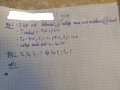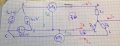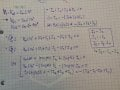# balanced delta connected voltage source with negative sequence and an unbalanced wye connected load (with neutral line)

#### Neophyte_2.0

Joined Aug 21, 2019
14
So given is that you have a balanced delta connected voltage source with negative sequence and an unbalanced wye connected load (with neutral line). Al the loads are given , the neutral current is given and the first phase voltage of the soure as Vp=Vm<10°. Asked: Ia,Ib and Ic and the Power measured by both wattmeters.

So I found 2 equations by doing mesh analysis and 1 equation as KCL in the neutral point (for 3 unkowns). But my last equation: 9,02+j4,53= -(Ia+Ib+Ic)= -(Ia-Ic-Ia+Ic)=0 so I wonder what I did wrong ? Thank you#### WBahn

Joined Mar 31, 2012
27,885
You show a non-zero neutral current coming in from the right. But where is that current coming from? What is the complete circuit that it is a part of?

•MrAl